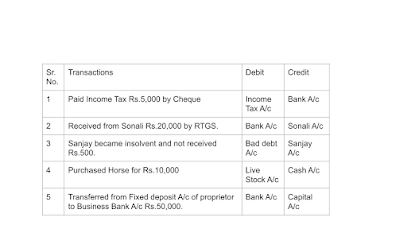# Journal Entries Solution With Explanations and Calculations of Standard 11th Maharashtra State Board. All Textbook Practical Problems are Solved. Students can ask there doubts in comment box.  Below are the Practical Problems click on the problem to get solution with explanations and calculations. PRACTICAL PROBLEMS   1. Journalize the following transactions in the books of Anand General Merchants.    2. Journalize the following transactions in the books of Gajanan.   3. Journalize the following transactions in the books of Ashok General Stores.      4. Journalize the following transactions in the books of Sanjay General Stores.      5. Journalize the following transactions in the books of Kunal Stores.    (adsbygoogle = window.adsbygoogle || []).push({});  6. Journalize the following transactions in the books of Nina General Stores.    7. Journalize the following transactions in the books of Varun.    8. Journalize the following transactions in the books of Dhoni Auto Car Centre.   9. Journalize the following transactions in the books of Hero Enterprises.   10. Journalize the following transactions in the books of Harbhajan & Co. for the month of 1st April 2019.  Q.9. Calculate the following. 1) Purchased Motor Car from Tata & Co. worth Rs.2,00,000 at 18% GST. Find out GST amount. = 2,00,000 *18/100 =36,000 The GST amount is Rs.36,000.   2) Paid Transport charges Rs.10,000 @5% GST. Calculate CGST & SGST. CGST @2.5% =10,000 *2.5/100 = 250 SGST @2.5% = 10,000 *2.5/100 = 250   3) Bought Goods from Ranjan Rs.10,000 @5% GST and 10% cash Discount. Calculate cash discount. Cash discount =10,000 *10/100 = 1,000 GST @5%= 1,000 *5/100 = 50 Add both the Amount 1,000 + 50 = 1,050   4)Received cheque of Rs.90,000 from Kiran in full settlement of his account Rs.1,00,000/-. Calculate discount rate. List amount =1,00,000 and received amount =90,000 "Discount amount = list amt - received amt"   = 1,00,000 - 90,000   = 10,000   "Rate of Discount = Discount/List amt *100"  =10,000/1,00,000 *100  =10%   5)Sold goods of Rs.1,00,000 at 10%. Trade Discount and 10% Cash discount to Ram and received 50% amount by cheque. Calculate the amount of cheque received. T.D @10% = 1,00,000 *10/100 = 10,000 C.D @10% = 90,000 *10/100 = 9,000 50% by Cheque = 81,000 *50/100 = 40,500   Q.10 Complete the following table.Journal Entries   Chapter 4, Ledger Solution

1.It is too help full ..... Thank you 😊😊😊😊❣️

2.Thank you so much.

3.Google Gemini: A New Era in AI by Google Google has unveiled Gemini, its largest language model. Dubbed Gemini 1.0, this AI marks the dawn of a new era, introduced by Google CEOs Sundar Pichai and Demis Hassabis. Gemini, a multimodal AI, is pre-trained and fine-tuned on various modalities, excelling in programming and boasting 2x speed compared to its predecessor. Expected to integrate into Google Search, Chrome, and beyond, Gemini promises enhanced performance across diverse inputs.

# Student Reviews

This is very helpful for getting a solution.

I like all your posts. You have done really good work. Thank you for the information you provide; it helped me a lot. I hope to have many more entries or so from you.

The answers are so perfect and proper 🌝

Nice explanation in full detail is very helpful.

It's very easy to learn.

Privacy Statement: We respect student privacy and do not share any personal information. The reviews shared are voluntarily provided by students to showcase their genuine appreciation for our educational content.